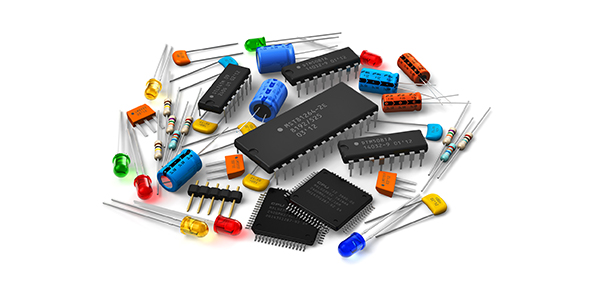# Random Quiz On Electronics Device!

15 Questions | Total Attempts: 50SettingsThere are different types of electronic devices each having a specific purpose over the other. Having covered the topic on electronic devices you are now able to point out different devices. Test your knowledge on different electronic devices and how they operate. Try out the random quiz below and see for yourself.

• 1.
What is the total phase shift requirement around the feedback loop for a phase-shift oscillator
• A.

90

• B.

180

• C.

180

• D.

360

• 2.
The start up gain of an oscillator must be which one
• A.

Equal to

• B.

Less than

• C.

Greater than

• D.

360None of the above

• 3.
At series resonance the impedance of a crystal is
• A.

Minimum

• B.

Maximum

• C.

Equal

• D.

Equal

• 4.
For frequencies up to 1 MHz Oscillator is the most widely used type of sinusoidal RC oscillator
• A.

Wien bridge

• B.

Phase shift

• C.

Colpitts

• D.

None of the above

• 5.
The feedback signal in which oscillator is derived from a capacitive voltage divider in the LC circuit
• A.

Hartley

• B.

Armstrong

• C.

Colpitts

• D.

Wein bridge

• 6.
Which of the following improvements is  a result of the negative feedback in a circuit
• A.

Lower output impedance

• B.

Reduced noise

• C.

More linear operation

• D.

All of the above}

• 7.
The feedback signal in which  oscillator is derived from an inductive voltage divider in the LC circuit
• A.

Armstrong

• B.

Hartley

• C.

Colpitts

• D.

Wein bridge

• 8.
The attenuation of the three-section RC feedback phase-shift oscillator is
• A.

One divided by nine

• B.

One divided by twenty nine

• C.

One divided by thirty

• D.

One divide by seven

• 9.
At resonance the tuned circuits offers
• A.

Low impedance

• B.

Both high and low impedence

• C.

High impedance

• D.

None of the above

• 10.
The ratio of difference in slope at beginning and end of sweep to the intial value of slope i
• A.

Displacement error

• B.

Transmission error

• C.

Both

• D.

Sweep speed error

• 11.
The maximum difference between the actual sweep voltage and linear sweep which passes through the beginning and end points of the actual sweep is
• A.

Frequency error

• B.

Transmission error

• C.

Sweep speed error

• D.

Displacement error

• 12.
To have a good linearity which type of ramp generator is used
• A.

Bootstrap generator

• B.

UJT type circuit

• C.

Any ramp circuit

• D.

A miller integrating type circuit

• 13.
The following ramp circuit is used in television receivers for display
• A.

Ujt generator

• B.

Boot strap

• C.

Voltage time base generator

• D.

None of the above

• 14.
Linear ramp generators are also known as sweep generators
• A.

Might be

• B.

False

• C.

True

• D.

None of the above

• 15.
The period during which voltage increases linearly is called
• A.

Time period

• B.

Sweep time

• C.

Duty cycle

• D.

Frequency

Related TopicsBack to top